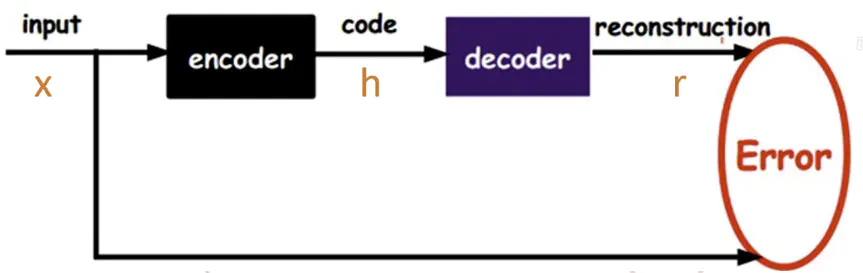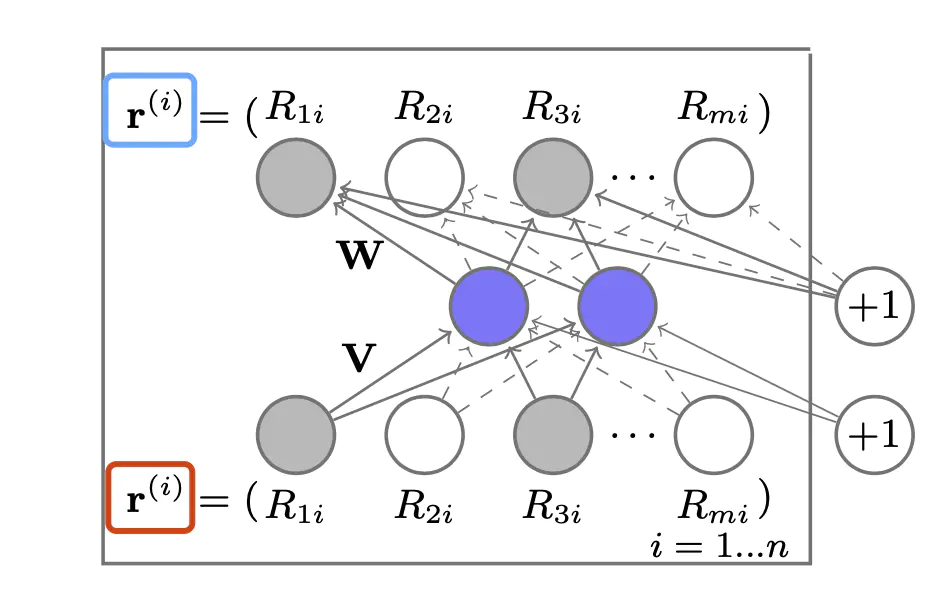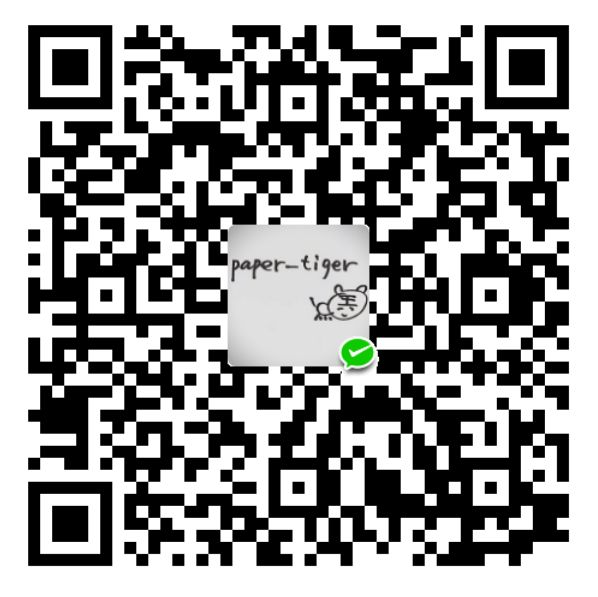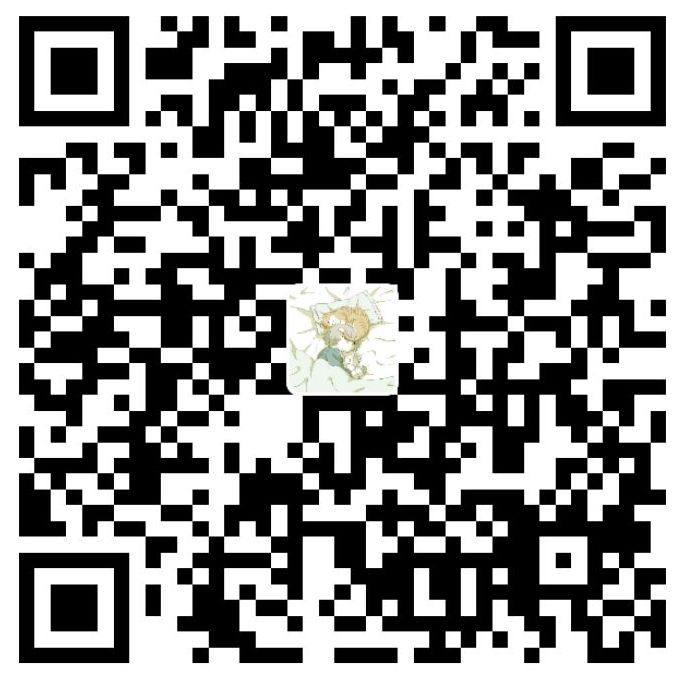### 简介

$AutoRec$ 模型是由澳大利亚国立大学在2015年提出的，它将自编码器($AutoEncoder$)的思想与协同过滤($Collaborative Filter$)的思想结合起来，提出了一种单隐层的简单神经网络推荐模型。可以说这个模型的提出，拉开了使用深度学习解决推荐系统问题的序幕，为复杂深度学习网络的构建提供了思路。

### 一、自动编码器AutoEncoder

#### 1.1 自编码器的介绍$$min \mathop{\sum}\limits_{r \in S} ||r-g(h)||^2_2$$

Q1：输出向量与输入向量误差最小有什么意义？直接使用原始输入向量不行吗？为什么需要编码和解码这些操作？

A1：比如协同过滤中，每个用户对每个物品不可能都评过分，即当数据规模较大时，共现矩阵是一个巨大的稀疏矩阵，该矩阵存在大量的缺失值。故将初始向量通过已训练好的AutoEncoder模型得到的输出向量会因为网络权重的作用而自动填补一些缺失值，与输入向量有所区别。

Q2：既然共现矩阵稀疏，存在大量缺失值，怎么处理缺失值？训练的效果会好吗？

A2：缺失值的处理可以尝试用0代替，在训练过程中，不同输入向量的不同缺失值相互弥补了输出向量的空缺。个人理解是某些输入向量的训练集中在某些权值上，而另一些与其缺失值不同的输入向量的训练集中在另一些权值上。

#### 1.2 自编码器与前馈神经网络的比较：

• 自编码器是前馈神经网络的一种，最开始主要用于数据的降维以及特征的抽取，随着技术的不断发展，现在也被用于生成模型中，可用来生成图片等。
• 前馈神经网络是有监督学习，其需要大量的标注数据；自编码器是无监督学习，数据不需要标注因此较容易收集。
• 前馈神经网络在训练时主要关注输出层的数据以及错误率；而自编码器可能更多的关注中间隐层的结果。

### 二、AutoRec

$AutoRec$ 模型跟多层感知机类似，是一个标准的3层全连接神经网络，只不过它结合了自编码器和协同过滤的思想。再确切一点说，$AutoRec$ 模型就是一个标准的自编码器结构，它的基本原理是利用协同过滤中的共现矩阵，完成物品向量或者用户向量的自编码，再利用自编码结果得到用户对所有物品的评分，结果通过排序之后就可以用于物品推荐。

#### 2.1 模型结构$$h(r;\theta) = f(W·g(Vr+\mu)+b)$$

#### 2.2 损失函数

$$\mathop{min}\limits_{\theta}\sum^n_{i=1}||r^{(i)}-h(r^{(i)};\theta)||^2_2+\frac{\lambda}{2}(||W||^2_2+||V||^2_2)$$

#### 2.3 基于AutoRec的推荐过程

##### Item-AutoRec
• 依次输入物品 $i$ 的评分向量 $r^{(i)}$，得到模型输出的预测评分向量 $h(r^{(i)};\theta)$；
• 遍历所有物品预测评分向量的第 $u$ 维，得到用户 $u$ 对所有物品的评分，进行排序之后得到用户 $u$ 的推荐列表。
##### User-AutoRec
• 依次输入用户 $u$ 的评分向量 $r^{(u)}$，得到模型输出的预测评分向量 $h(r^{(u)};\theta)$；
• 进行排序得到用户 $u$ 的推荐列表。

### 四、总结

$AutoRec$ 模型是深度学习方法用于推荐系统中的开山之作，它仅仅使用了一个单隐层的自编码器来泛化用户和物品评分，使模型具有一定的泛化和表达能力。但是由于模型过于简单，也让它在实际使用中显得表征能力不足。微信支付宝